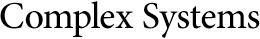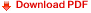## Chaotic Optimization and the Construction of Fractals: Solution of an Inverse ProblemGiorgio Mantica
Department of Physics, Atlanta University, Atlanta, GA 30314, USA
and
School of Physics, Georgia Institute of Technology,
Atlanta, GA 30332-0430, USA

Alan Sloan
School of Mathematics, Georgia Institute of Technology,
Atlanta, GA 30332, USA
and
Iterated Systems Incorporated, 5550 P'tree Parkway, Suite 545,
Atlanta, GA 30092, USA

#### Abstract

An inverse problem in fractal set construction is introduced in this paper, according to the theory of iterated function systems (IFS). This theory allows the construction of a class of fractals depending on a finite number of parameters. Finding a set of parameters which reconstructs a given fractal is the goal of the inverse problem. As the solution of the inverse problem generally involves a compression of the information encoded in the fractal, complexity theory is here applied. In particular, we define the IFS-entropy to characterize the class of fractals for which the problem can be profitably solved.

The inverse problem can be reduced to the minimization of a suitable function in parameter space. We describe a new algorithm to obtain a reliable minimum solution, which originates from the theory of dynamical systems. We suggest that this algorithm should greatly improve simulated thermal annealing à la Kirkpatrick-Szu when a metric structure can be given to an optimization problem.